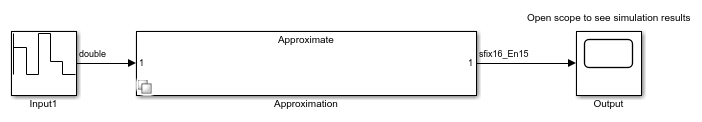# approximate

Class: FunctionApproximation.LUTSolution
Package: FunctionApproximation

Generate a Lookup Table block or lookup table as a MATLAB function from a `FunctionApproximation.LUTSolution`

## Syntax

``approximate(solution)``
``approximate(solution,'Name',fileName)``
``approximate(solution,'Name',fileName,'Path',filePath)``

## Description

example

````approximate(solution)` generates either a Simulink® model containing a subsystem made up of the Lookup Table block, or a lookup table as a MATLAB® function, depending on the `ApproximateSolutionType` property of the `FunctionApproximation.Options` object. Data and breakpoints of the generated lookup table are specified by the `FunctionApproximation.LUTSolution` object, `solution`. The generated Lookup Table block is surrounded with Data Type Conversion blocks.```
````approximate(solution,'Name',fileName)` generates a lookup table as a MATLAB function with the name of the generated .m script specified by `fileName`. This option is only available when the `ApproximateSolutionType` property of `FunctionApproximation.Options` is set to `MATLAB`.```
````approximate(solution,'Name',fileName,'Path',filePath)` generates a lookup table as a MATLAB function with the file path for the generated .m script specified by `filePath`. This option is only available when the `ApproximateSolutionType` property of `FunctionApproximation.Options` is set to `MATLAB`.```

## Input Arguments

expand all

The solution to generate a lookup table from, specified as a `FunctionApproximation.LUTSolution` object.

File name for generated MATLAB function, specified as a character array. If no custom file name is specified, a time stamp is used to generate a unique file name. For example, `approximateFunction_20210617T111033122.m`.

Example: `approximate(solution,'Name','myLUT')`

Data Types: `char`

File path for generated MATLAB function, specified as a character array. If no custom file path name is specified, the current working directory is used.

Example: `approximate(solution,'Name','myLUT','Path','C:\Users\myPath')`

Data Types: `char`

## Examples

expand all

Create a `FunctionApproximation.Problem` object defining the function you want to approximate.

```problem = FunctionApproximation.Problem('tanh') ```
```problem = 1x1 FunctionApproximation.Problem with properties: FunctionToApproximate: @(x)tanh(x) NumberOfInputs: 1 InputTypes: "numerictype(1,16,12)" InputLowerBounds: -8 InputUpperBounds: 8 OutputType: "numerictype(1,16,15)" Options: [1x1 FunctionApproximation.Options] ```

Use default values for all other options. Approximate the `tanh` function using the `solve` method.

```solution = solve(problem) ```
```Searching for fixed-point solutions. | ID | Memory (bits) | Feasible | Table Size | Breakpoints WLs | TableData WL | BreakpointSpecification | Error(Max,Current) | | 0 | 64 | 0 | 2 | 16 | 16 | EvenSpacing | 7.812500e-03, 7.229091e-01 | | 1 | 1248 | 1 | 76 | 16 | 16 | EvenSpacing | 7.812500e-03, 4.368265e-03 | | 2 | 1232 | 1 | 75 | 16 | 16 | EvenSpacing | 7.812500e-03, 4.439035e-03 | | 3 | 944 | 1 | 57 | 16 | 16 | EvenSpacing | 7.812500e-03, 7.780470e-03 | | 4 | 928 | 1 | 56 | 16 | 16 | EvenSpacing | 7.812500e-03, 6.110240e-03 | | 5 | 656 | 0 | 39 | 16 | 16 | EvenSpacing | 7.812500e-03, 1.678519e-02 | | 6 | 640 | 0 | 38 | 16 | 16 | EvenSpacing | 7.812500e-03, 1.660649e-02 | | 7 | 784 | 1 | 47 | 16 | 16 | EvenSpacing | 7.812500e-03, 7.743777e-03 | | 8 | 704 | 1 | 42 | 16 | 16 | EvenSpacing | 7.812500e-03, 7.805676e-03 | | 9 | 672 | 1 | 40 | 16 | 16 | EvenSpacing | 7.812500e-03, 7.809550e-03 | | 10 | 368 | 0 | 21 | 16 | 16 | EvenSpacing | 7.812500e-03, 4.855583e-02 | | 11 | 512 | 0 | 30 | 16 | 16 | EvenSpacing | 7.812500e-03, 2.773526e-02 | | 12 | 592 | 0 | 35 | 16 | 16 | EvenSpacing | 7.812500e-03, 2.088471e-02 | | 13 | 624 | 0 | 37 | 16 | 16 | EvenSpacing | 7.812500e-03, 1.870074e-02 | | 14 | 384 | 1 | 12 | 16 | 16 | ExplicitValues | 7.812500e-03, 7.812317e-03 | | 15 | 384 | 0 | 12 | 16 | 16 | ExplicitValues | 7.812500e-03, 1.196141e-02 | | 16 | 384 | 1 | 12 | 16 | 16 | ExplicitValues | 7.812500e-03, 7.812317e-03 | Best Solution | ID | Memory (bits) | Feasible | Table Size | Breakpoints WLs | TableData WL | BreakpointSpecification | Error(Max,Current) | | 14 | 384 | 1 | 12 | 16 | 16 | ExplicitValues | 7.812500e-03, 7.812317e-03 | solution = 1x1 FunctionApproximation.LUTSolution with properties: ID: 14 Feasible: "true" ```

Generate a Simulink™ subsystem containing a Lookup Table block approximating the `tanh` function.

```approximate(solution) ```## Version History

Introduced in R2018a